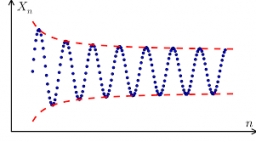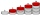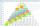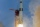# 15th term of AP

What is the 15th term; x1=1.5, d=4.5?

x15 =  64.5

### Step-by-step explanation:Did you find an error or inaccuracy? Feel free to write us. Thank you!## Related math problems and questions:

• Arithmetic progression 2The 3rd term of an Arithmetic progression is ten more than the first term, while the fifth term is 15 more than the second term. Find the sum of the 8th and 15th terms of the Arithmetic progression if the 7th term is seven times the first term.
• What is 10What is the 5th term, if the 8th term is 80 and common ratio r =1/2?
• Difference AP 4Calculate the difference of the AP if a1 = 0.5, a2 + a3 = -1.1
• Missing term 2What is the missing term for the Geometric Progression (GP) 3, 15, 75,__, 1875?
• Trapezium internal anglesA trapezium where AB is parallel to CD, has angle A : angle D = 4 :5, angle B = 3x-15 and angle C = 4x+20. Find angle A, B, C and D.
• HP - harmonic progressionDetermine the 8th term of the harmonic progression 2, 4/3, 1,…
• Trapezoid 15Area of trapezoid is 266. What value is x if bases b1 is 2x-3, b2 is 2x+1 and height h is x+4
• 10th termWhat is the 10th term of the Arithmetic Progression if x1=4 and d=5?
• Expression with powersIf x-1/x=5, find the value of x4+1/x4
• Third memberDetermine the third member of the AP if a4=93, d=7.5.
• AP membersWhat is the value of x2, x3, x4, x5…of the terms (of arithmetic progression) when x1 = 8 and x6 = 20?
• Arithmetic progressionIn some AP applies: 5a2 + 7a5 = 90 s3 = 12 Find the first member a =? and difference d = ?
• Find the 2Find the term independent of x in the expansion of (4x3+1/2x)8
• HP - harmonic progression 2Compute the 16th term of the HP if the 6th and 11th term of the harmonic progression are 10 and 18 respectively.
• Sequence 11What is the nth term of this sequence 1, 1/2, 1/3, 1/4, 1/5?
• Common differenceThe 4th term of an arithmetic progression is 6. If the sum of the 8th and 9th terms is -72, find the common difference.
• AlgebraIf x+y=5, find xy (find the product of x and y if x+y = 5)